# R: An introductory regression exercise

In this example, we will complete a linear regression in R using mtcars, one of the built-in R datasets.

1. help or ?
First, let us get acquainted with the mtcars dataset.
To do so, we look at the package description. We can do this by either using ‘?mtcars’ or ‘help(mtcars)’.
Note: this will print in the Help window, and has been pasted in to the Notebook for completeness.

``help(mtcars)``

mtcars {datasets}
R Documentation
Description

The data was extracted from the 1974 Motor Trend US magazine, and comprises fuel consumption and 10 aspects of automobile design and performance for 32 automobiles (1973–74 models).

Usage

mtcars

Format

A data frame with 32 observations on 11 variables.

[, 1] mpg Miles/(US) gallon
[, 2] cyl Number of cylinders
[, 3] disp Displacement (cu.in.)
[, 4] hp Gross horsepower
[, 5] drat Rear axle ratio
[, 6] wt Weight (1000 lbs)
[, 7] qsec 1/4 mile time
[, 8] vs V/S
[, 9] am Transmission (0 = automatic, 1 = manual)
[,10] gear Number of forward gears
[,11] carb Number of carburetors

Source

Henderson and Velleman (1981), Building multiple regression models interactively. Biometrics, 37, 391–411.

Examples

require(graphics)
pairs(mtcars, main = “mtcars data”)
coplot(mpg ~ disp | as.factor(cyl), data = mtcars,
panel = panel.smooth, rows = 1

Let’s look at the data itself.
The head function returns the first six lines of the data frame. You can also specify the number of lines to display as the second argument to head. For example, head(mtcars, 10) would display the first 10 lines.

``head(mtcars)``
```
mpg  cyl disp  hp  drat    wt    qsec  vs am gear carb
Mazda RX4         21.0  6  160  110  3.90  2.620  16.46  0   1   4   4
Mazda RX4 Wag     21.0  6  160  110  3.90  2.875  17.02  0   1   4   4
Datsun 710        22.8  4  108   93  3.85  2.320  18.61  1   1   4   1
Hornet 4 Drive    21.4  6  258  110  3.08  3.215  19.44  1   0   3   1
Hornet Sportabout 18.7  8  360  175  3.15  3.440  17.02  0   0   3   2
Valiant           18.1  6  225  105  2.76  3.460  20.22  1   0   3   1
```

3. dim
The dim function gives us the dimensions of the dataset.

``dim(mtcars)``
`` 32 11``

It looks like mtcars is a small dataset, containing information for 32 different car models.

4. summary (of data frame)
Let’s further familiarize ourselves with the data.
The summary of a dataframe returns the quartiles of each numeric column of the dataset.

``summary(mtcars)``
``````      mpg             cyl             disp             hp
Min.   :10.40   Min.   :4.000   Min.   : 71.1   Min.   : 52.0
1st Qu.:15.43   1st Qu.:4.000   1st Qu.:120.8   1st Qu.: 96.5
Median :19.20   Median :6.000   Median :196.3   Median :123.0
Mean   :20.09   Mean   :6.188   Mean   :230.7   Mean   :146.7
3rd Qu.:22.80   3rd Qu.:8.000   3rd Qu.:326.0   3rd Qu.:180.0
Max.   :33.90   Max.   :8.000   Max.   :472.0   Max.   :335.0
drat             wt             qsec             vs
Min.   :2.760   Min.   :1.513   Min.   :14.50   Min.   :0.0000
1st Qu.:3.080   1st Qu.:2.581   1st Qu.:16.89   1st Qu.:0.0000
Median :3.695   Median :3.325   Median :17.71   Median :0.0000
Mean   :3.597   Mean   :3.217   Mean   :17.85   Mean   :0.4375
3rd Qu.:3.920   3rd Qu.:3.610   3rd Qu.:18.90   3rd Qu.:1.0000
Max.   :4.930   Max.   :5.424   Max.   :22.90   Max.   :1.0000
am              gear            carb
Min.   :0.0000   Min.   :3.000   Min.   :1.000
1st Qu.:0.0000   1st Qu.:3.000   1st Qu.:2.000
Median :0.0000   Median :4.000   Median :2.000
Mean   :0.4062   Mean   :3.688   Mean   :2.812
3rd Qu.:1.0000   3rd Qu.:4.000   3rd Qu.:4.000
Max.   :1.0000   Max.   :5.000   Max.   :8.000  ``````

The following variables appear to be ordinal with a small subset of values, and could be treated as factors:
– cyl (number of cylinders): 4-8
– vs (V- or Standard engine): 0 or 1
– am (automatic or manual): 0 (automatic) or 1 (manual)
– gear (number of forward gears): 3-5
– carb (number fo carburators): 1-8

5. hist and boxplot
We can also look at boxplots and histograms of the variables.
par(mfrow) divides up the plot area so several plots can be displayed simultaneously. Specifically, par(mfrow=c(2,2)) divides the display area into a 2 x 2 grid, to display 4 plots.

``````par(mfrow=c(2,2))
hist(mtcars\$mpg, main = "Histogram of MPG")
boxplot(mtcars\$mpg, main = "Boxplot of MPG")``````
``````hist(mtcars\$wt, main = "Histogram of Weight")
boxplot(mtcars\$wt, main = "Boxplot of Weight")``````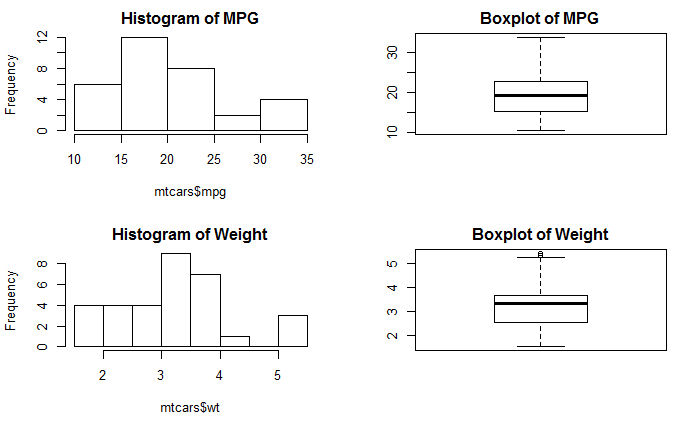6. boxplot (by factor)
Given that we have several variables that can be treated as factors, we may also want to look at conditional boxplots.
The ‘~’ notation is R notation for ‘function of’, so boxplot(mpg ~ gear) gives a boxplot for each category in gear.

``````par(mfrow=c(2,2))
boxplot(mpg ~ gear, data = mtcars, col = "light gray", main = "MPG by Gear")
boxplot(mpg ~ am, data = mtcars, col = "light gray", main = "MPG by A/M")``````
``````boxplot(mpg ~ vs, data = mtcars, col = "light gray", main = "MPG by V/S")
boxplot(mpg ~ carb, data = mtcars, col = "light gray", main = "MPG by Carb")``````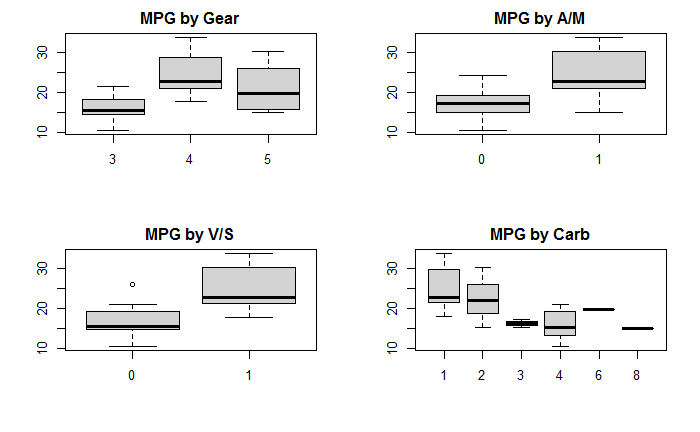7. pairs
We examine the correlation between the variables, first visually.
The pairs function plots pairwise scatterplots of the variables in the dataframe, helping to quickly see the relationship amongst the variables. The plot is a bit scrunched here in the Notebook, and therefore not as informative as it could be in larger-sized plot.

``pairs(mtcars)``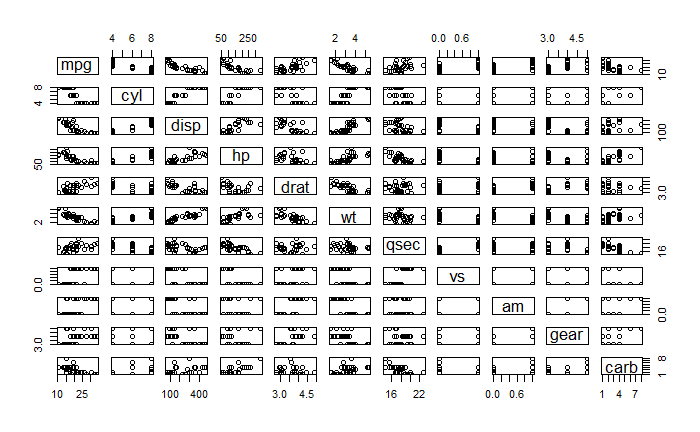8. corrplot
We could also create a fancier correlational plot using the corrplot library to visualize the corelation matrix.
You may need to install the corrplot library first, using Install Packages from the R Studio Tools menu, or the install function. Load the library into the current session using the library function: library(corrplot).

``````library(corrplot)
M <- cor(mtcars)  ## get the corelation matrix
corrplot.mixed(M) ## visualize the corelation matrix``````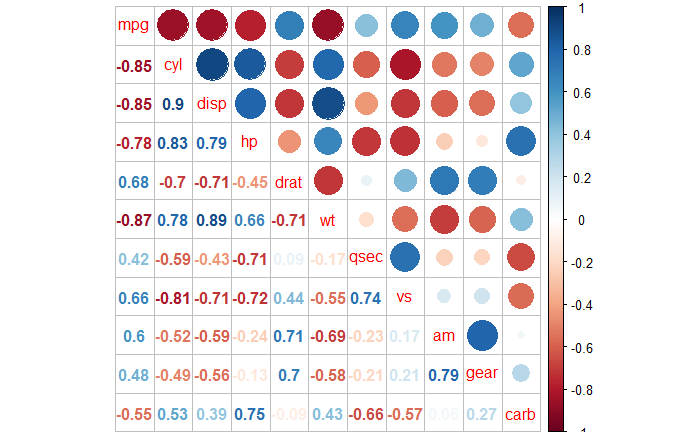From the correlational plots, we can see that many of our variables (or Xs) are highly correlated. We can also see that wt is highly correlated with mpg, which fits well with what we intuitively know about cars – that the mpg we get will depend on the weight of the car.

9. plot (scatterplots)
Before doing the regression, we may want to look at the scatterplots of mpg with the independent variables in more detail.
The text function adds labels, row numbers of the observations in this case.

``````plot(mpg ~ wt, data = mtcars)
text(mtcars\$wt, mtcars\$mpg, labels=seq(1:32), cex= 0.7, pos = 4)``````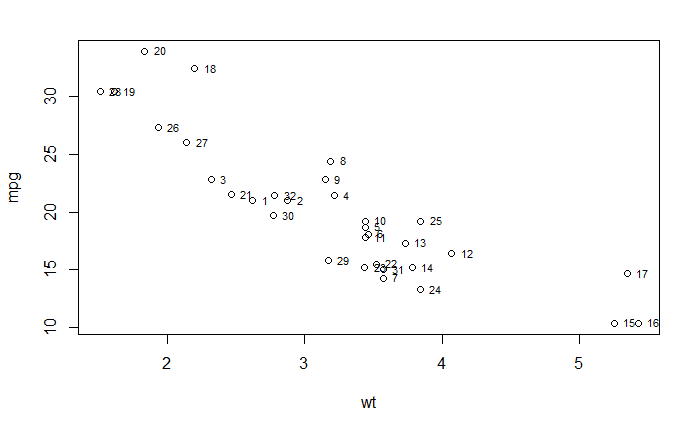``````plot(mpg ~ disp, data = mtcars)
text(mtcars\$disp, mtcars\$mpg, labels=seq(1:32), cex= 0.7, pos = 4)``````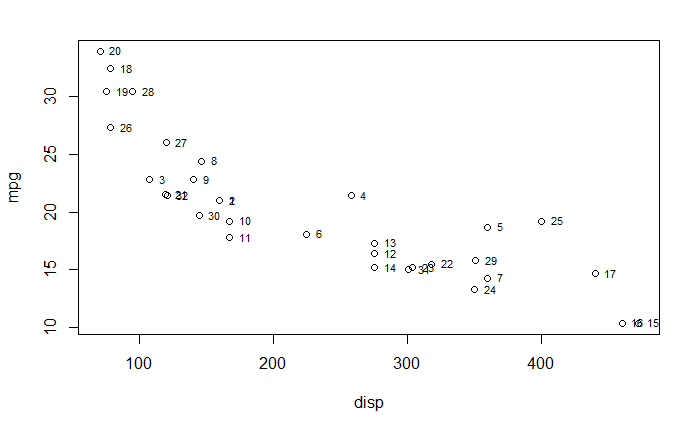``````plot(wt ~ disp, data = mtcars)
text(mtcars\$disp, mtcars\$wt, labels=seq(1:32), cex= 0.7, pos = 4)``````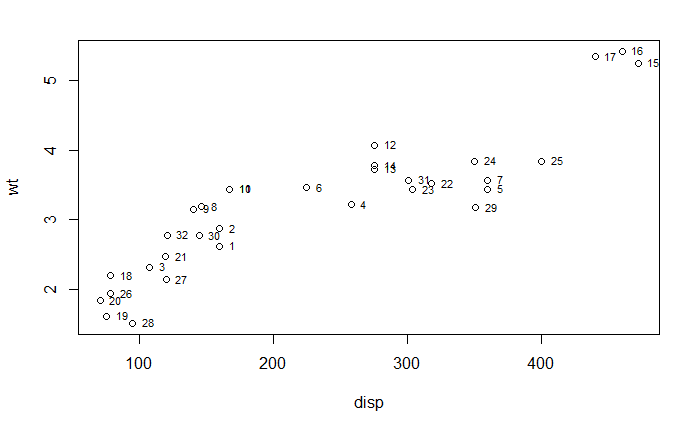These three scatterplots confirm what we learned from the correlational plots – both wt and disp are highly correlated with mpg and with each other.

10. lm
After having familiarized ourselves with the data, we can begin answering questions that might interest us; for example: how does mpg vary with other car attributes?

To answer the question, we begin with the simplest regression model – one with a single predictor. We choose wt as the predictor as it is both highly correlated with mpg and we inutitively expect the weight of a car to have a direct impact on mpg.
The R function lm – for linear model – is the function we use for linear regression. The summary function (on lm results) provides a summary of the regression model, including the co-efficients, p-values and R2.

``````m1 <- lm(mpg ~ wt, data = mtcars)
summary(m1)``````
``````
Call:
lm(formula = mpg ~ wt, data = mtcars)

Residuals:
Min      1Q  Median      3Q     Max
-4.5432 -2.3647 -0.1252  1.4096  6.8727

Coefficients:
Estimate Std. Error t value Pr(>|t|)
(Intercept)  37.2851     1.8776  19.858  < 2e-16 ***
wt           -5.3445     0.5591  -9.559 1.29e-10 ***
---
Signif. codes:  0 ‘***’ 0.001 ‘**’ 0.01 ‘*’ 0.05 ‘.’ 0.1 ‘ ’ 1

Residual standard error: 3.046 on 30 degrees of freedom
Multiple R-squared:  0.7528,    Adjusted R-squared:  0.7446
F-statistic: 91.38 on 1 and 30 DF,  p-value: 1.294e-10``````

Just the weight of the car, wt, explains about 74% of the variance in mpg in our sample of cars. We have seen from the variable scatterplots and the correlational plots that disp is highly correlated with wt. So the next variable to add would be either cyl or hp, both of which are also highly correlated with each other. Let’s begin with testing out cyl.

``````m2 <- lm(mpg ~ wt + cyl, data = mtcars)
summary(m2)``````
``````
Call:
lm(formula = mpg ~ wt + cyl, data = mtcars)

Residuals:
Min      1Q  Median      3Q     Max
-4.2893 -1.5512 -0.4684  1.5743  6.1004

Coefficients:
Estimate Std. Error t value Pr(>|t|)
(Intercept)  39.6863     1.7150  23.141  < 2e-16 ***
wt           -3.1910     0.7569  -4.216 0.000222 ***
cyl          -1.5078     0.4147  -3.636 0.001064 **
---
Signif. codes:  0 ‘***’ 0.001 ‘**’ 0.01 ‘*’ 0.05 ‘.’ 0.1 ‘ ’ 1

Residual standard error: 2.568 on 29 degrees of freedom
Multiple R-squared:  0.8302,    Adjusted R-squared:  0.8185
F-statistic: 70.91 on 2 and 29 DF,  p-value: 6.809e-12``````

Together wt and cyl explain 82% of the variance in mpg – not bad. How does the wt + hp model compare?

``````m2a <- lm(mpg ~ wt + hp, data = mtcars)
summary(m2a)``````
``````
Call:
lm(formula = mpg ~ wt + hp, data = mtcars)

Residuals:
Min     1Q Median     3Q    Max
-3.941 -1.600 -0.182  1.050  5.854

Coefficients:
Estimate Std. Error t value Pr(>|t|)
(Intercept) 37.22727    1.59879  23.285  < 2e-16 ***
wt          -3.87783    0.63273  -6.129 1.12e-06 ***
hp          -0.03177    0.00903  -3.519  0.00145 **
---
Signif. codes:  0 ‘***’ 0.001 ‘**’ 0.01 ‘*’ 0.05 ‘.’ 0.1 ‘ ’ 1

Residual standard error: 2.593 on 29 degrees of freedom
Multiple R-squared:  0.8268,    Adjusted R-squared:  0.8148
F-statistic: 69.21 on 2 and 29 DF,  p-value: 9.109e-12``````

The two models are pretty comparable…the R2 for the two models are very close, and both predictors are significant…which model to choose?

We could also try adding a third variable to the model. Since there are only 32 samples in the dataset, 3 predictors is probably enough, following the rule of thumb of ~10 observations per predictor. Below, we add am…you can play with others. Since the predictor variables are highly correlated, the results will be similar.

``````m3 <- lm(mpg ~ wt + cyl + am, data = mtcars)
summary(m3)``````
``````
Call:
lm(formula = mpg ~ wt + cyl + am, data = mtcars)

Residuals:
Min      1Q  Median      3Q     Max
-4.1735 -1.5340 -0.5386  1.5864  6.0812

Coefficients:
Estimate Std. Error t value Pr(>|t|)
(Intercept)  39.4179     2.6415  14.923 7.42e-15 ***
wt           -3.1251     0.9109  -3.431  0.00189 **
cyl          -1.5102     0.4223  -3.576  0.00129 **
am            0.1765     1.3045   0.135  0.89334
---
Signif. codes:  0 ‘***’ 0.001 ‘**’ 0.01 ‘*’ 0.05 ‘.’ 0.1 ‘ ’ 1

Residual standard error: 2.612 on 28 degrees of freedom
Multiple R-squared:  0.8303,    Adjusted R-squared:  0.8122
F-statistic: 45.68 on 3 and 28 DF,  p-value: 6.51e-11``````

We see from the summary for model m3 that am does not add any predictive power to the model – the R2 is essentially unchanged, and am is not significant (the p-value is 0.89) – if wt and cyl are already included in the model.

11. vif
Given the high degree of correlation – or multi-collinearity – of the predictor variables, it is probably a good idea to check the VIF (variance inflation factor).
You may need to install the car (companion to applied regression) package, and load the library.

``````library(car)
vif(m2); vif(m2a); vif(m3)``````
``````      wt      cyl
2.579312 2.579312
wt       hp
1.766625 1.766625
wt      cyl       am
3.609011 2.584066 1.924955 ``````

Depending on the purpose of the model, VIFs of 10 or even higher are okay. The model VIFs which are all below 3.7 indicate that they do not have excessive multi-collinearity. However, since am does not add any predictive power, there is no need to consider m3 any further.

12. Diagnostics plots for lm
How do we choose between m2 (mpg ~ wt + cyl) and m2a (mpg ~ wt + hp)? One way is to see which model has better diagnostics.
Note that diagnostics tell you how well the model fits the underlying assumptions of linear regression. So it is an important step for all regression models.

``````par(mfrow=c(2,2))
plot(m2)``````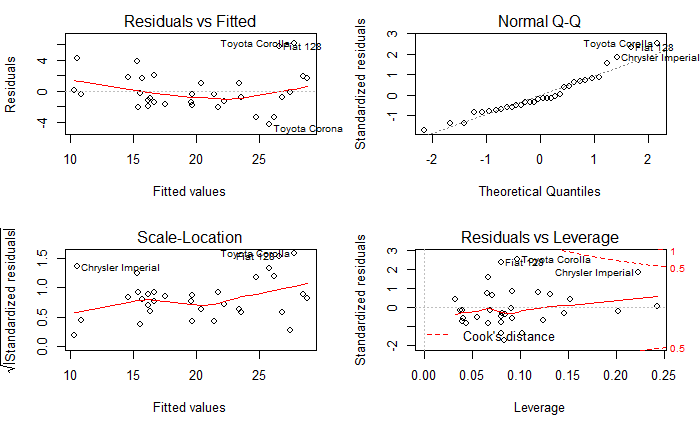``````par(mfrow = c(2,2))
plot(m2a)``````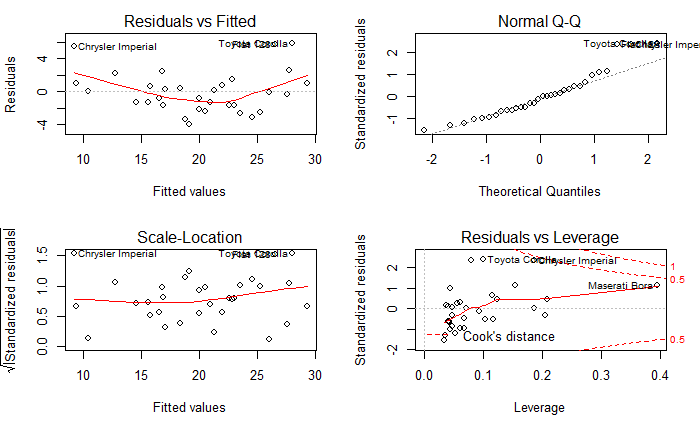Comparing the diagnostics for m2 (mpg ~ wt + cyl) and m2a (mpg ~ wt + hp), we see that the diagnostics are a little bit better for m2a. The residual plots, which test the assumption that the error term (residuals) are random, are comparable – may be marginally better for m2a, while the qq plot, which tests the assumption of normality of the residuals,is slightly better than for m2a than m2. The scale-location plot, which is a test for residuals being independent of predictors, might be a little bit better for m2a, and the leverage plots (which measure the influence of individual observations on the model) are comparable. All in all, based on our sample set, m2a might be a slightly better model choice.

Next steps
R packages (stats, corrplot, car, MASS) make developing descriptive and predictive linear regression models relatively simple. We developed a regression model using a very small subset of R funtionality. For example, for a data set with a greater number of predictors and observations, we could do a stepwise regression using the step function in the stats package or stepAIC in MASS package. We can also fit polynomials and look for interactions between variables. For more complex statistical modeling, R has packages like gam (general additive models); glm (generalized linear models) and nlme (multi-level modeling).